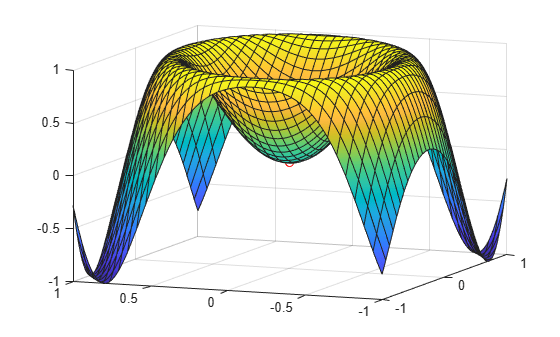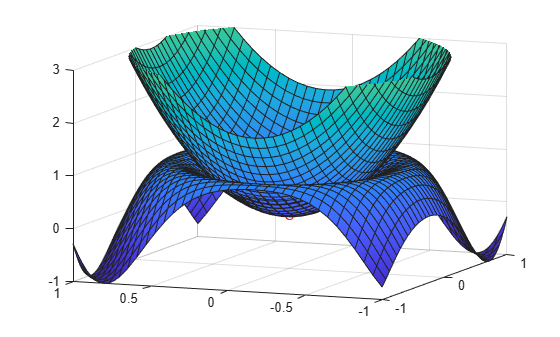# Find Extremum of Multivariate Function and Its Approximation

This example shows how to find the extremum of a multivariate function and its approximation near the extremum point. This example uses symbolic matrix variables to represent the multivariate function and its derivatives. Symbolic matrix variables are available starting in R2021a.

Consider the multivariate function $\mathit{f}\left(\mathbit{x}\right)=\mathrm{sin}\left({\mathbit{x}}^{\mathit{T}}\mathbit{A}\text{\hspace{0.17em}}\mathbit{x}\right)$, where $x$ is a 2-by-1 vector and $A$ is a 2-by-2 matrix. To find a local extremum of this function, compute a root of the derivative of $f\left(x\right)$. In other words, find the solution of the derivative $\nabla f\left({x}_{0}\right)=0$.

### Create Function and Find Its Derivative

Create the vector $x$ and the matrix $A$ as symbolic matrix variables. Define the function $\mathit{f}\left(\mathbit{x}\right)=\mathrm{sin}\left({\mathbit{x}}^{\mathit{T}}\mathbit{A}\text{\hspace{0.17em}}\mathbit{x}\right)$.

```syms x [2 1] matrix syms A [2 2] matrix f = sin(x.'*A*x)```
`f = $\mathrm{sin}\left({x}^{\mathrm{T}} A x\right)$`

Compute the derivative `D` of the function $f\left(x\right)$ with respect to the vector $x$. The derivative `D` is displayed in compact matrix notation in terms of $x$ and $A$.

`D = diff(f,x)`
`D = $\mathrm{cos}\left({x}^{\mathrm{T}} A x\right) \left({x}^{\mathrm{T}} A+{x}^{\mathrm{T}} {A}^{\mathrm{T}}\right)$`

### Convert `symmatrix` Objects to `sym` Objects

The symbolic matrix variables `x`, `A`, `f`, and `D` are `symmatrix` objects. These objects represent matrices, vectors, and scalars in compact matrix notation. To show the components of these variables, convert the `symmatrix` objects to `sym` objects using `symmatrix2sym`.

`xsym = symmatrix2sym(x)`
```xsym =  $\left(\begin{array}{c}{x}_{1}\\ {x}_{2}\end{array}\right)$```
`Asym = symmatrix2sym(A)`
```Asym =  $\left(\begin{array}{cc}{A}_{1,1}& {A}_{1,2}\\ {A}_{2,1}& {A}_{2,2}\end{array}\right)$```
`fsym = symmatrix2sym(f)`
`fsym = $\mathrm{sin}\left({x}_{1} \left({A}_{1,1} {x}_{1}+{A}_{1,2} {x}_{2}\right)+{x}_{2} \left({A}_{2,1} {x}_{1}+{A}_{2,2} {x}_{2}\right)\right)$`
`Dsym = symmatrix2sym(D)`
`Dsym = $\left(\begin{array}{cc}\mathrm{cos}\left({x}_{1} \left({A}_{1,1} {x}_{1}+{A}_{1,2} {x}_{2}\right)+{x}_{2} \left({A}_{2,1} {x}_{1}+{A}_{2,2} {x}_{2}\right)\right) \left(2 {A}_{1,1} {x}_{1}+{A}_{1,2} {x}_{2}+{A}_{2,1} {x}_{2}\right)& \mathrm{cos}\left({x}_{1} \left({A}_{1,1} {x}_{1}+{A}_{1,2} {x}_{2}\right)+{x}_{2} \left({A}_{2,1} {x}_{1}+{A}_{2,2} {x}_{2}\right)\right) \left({A}_{1,2} {x}_{1}+{A}_{2,1} {x}_{1}+2 {A}_{2,2} {x}_{2}\right)\end{array}\right)$`

### Substitute Numeric Values and Find the Minimum

Suppose you are interested in the case where the value of $A$ is `[2 -1; 0 3]`. Substitute this value into the function `fsym`.

`fsym = subs(fsym,Asym,[2 -1; 0 3])`
`fsym = $\mathrm{sin}\left(3 {{x}_{2}}^{2}+{x}_{1} \left(2 {x}_{1}-{x}_{2}\right)\right)$`

Substitute the value of $A$ into the derivative `Dsym`

`Dsym = subs(Dsym,Asym,[2 -1; 0 3])`
`Dsym = $\left(\begin{array}{cc}\mathrm{cos}\left(3 {{x}_{2}}^{2}+{x}_{1} \left(2 {x}_{1}-{x}_{2}\right)\right) \left(4 {x}_{1}-{x}_{2}\right)& -\mathrm{cos}\left(3 {{x}_{2}}^{2}+{x}_{1} \left(2 {x}_{1}-{x}_{2}\right)\right) \left({x}_{1}-6 {x}_{2}\right)\end{array}\right)$`

Then, apply the symbolic function `solve` to get a root of the derivative.

```[xmin,ymin] = solve(Dsym,xsym,'PrincipalValue',true); x0 = [xmin; ymin]```
```x0 =  $\left(\begin{array}{c}0\\ 0\end{array}\right)$```

Plot the function $f\left(x\right)$ together with the extremum solution ${x}_{0}$. Set the plot interval to $-1<{x}_{1}<1$ and $-1<{x}_{2}<1$ as the second argument of `fsurf`. Use `fplot3` to plot the coordinates of the extremum solution.

```fsurf(fsym,[-1 1 -1 1]) hold on fplot3(xmin,ymin,subs(fsym,xsym,x0),'ro') view([-68 13])```### Approximate Function Near Its Minimum

You can approximate a multivariate function around a point ${x}_{0}$ with a multinomial using the Taylor expansion.

`$f\left(x\right)\approx f\left({x}_{0}\right)+\nabla f\left({x}_{0}\right)\cdot \left(x-{x}_{0}\right)+\frac{1}{2}\left(x-{x}_{0}{\right)}^{T}\phantom{\rule{0.2777777777777778em}{0ex}}H\left(f\left({x}_{0}\right)\right)\phantom{\rule{0.2777777777777778em}{0ex}}\left(x-{x}_{0}\right)$`

Here, the term $\nabla f\left({x}_{0}\right)$ is the gradient vector, and $H\left(f\left({x}_{0}\right)\right)$ is the Hessian matrix of the multivariate function $\mathit{f}\left(\mathbit{x}\right)$ calculated at ${x}_{0}$.

Find the Hessian matrix and return the result as a symbolic matrix variable.

`H = diff(f,x,x.')`
`H = $-\mathrm{sin}\left({x}^{\mathrm{T}} A x\right) \left({A}^{\mathrm{T}} x+A x\right) \left({x}^{\mathrm{T}} A+{x}^{\mathrm{T}} {A}^{\mathrm{T}}\right)+\mathrm{cos}\left({x}^{\mathrm{T}} A x\right) \left({A}^{\mathrm{T}}+A\right)$`

Convert the Hessian matrix $H\left(f\left({x}_{0}\right)\right)$ to the `sym` data type, which represents the matrix in its component form. Use `subs` to evaluate the Hessian matrix for $A$` = [2 -1; 0 3]` at the minimum point ${x}_{0}$.

```Hsym = symmatrix2sym(H); Hsym = subs(Hsym,Asym,[2 -1; 0 3]); H0 = subs(Hsym,xsym,x0)```
```H0 =  $\left(\begin{array}{cc}4& -1\\ -1& 6\end{array}\right)$```

Evaluate the gradient vector $\nabla f\left({x}_{0}\right)$ at ${x}_{0}$.

`D0 = subs(Dsym,xsym,x0)`
`D0 = $\left(\begin{array}{cc}0& 0\end{array}\right)$`

Compute the Taylor approximation to the function near its minimum.

`fapprox = subs(fsym,xsym,x0) + D0*(xsym-x0) + 1/2*(xsym-x0).'*H0*(xsym-x0)`
```fapprox =  ${x}_{1} \left(2 {x}_{1}-\frac{{x}_{2}}{2}\right)-{x}_{2} \left(\frac{{x}_{1}}{2}-3 {x}_{2}\right)$```

Plot the function approximation on the same graph that shows $f\left(x\right)$ and ${x}_{0}$.

```hold on fsurf(fapprox,[-1 1 -1 1]) zlim([-1 3])```